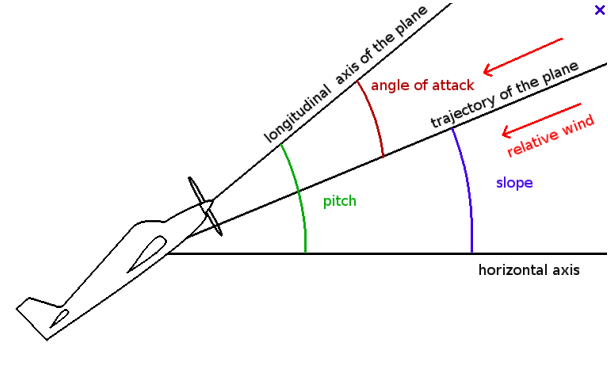Servers by jDrones# I search to collect the angle of attack or the slope, is it possible?

Hi , I have a major question , it could be the last if it works !Actually we have access to the pitch , but I also need the angle of attack, is it possible ?You can calculate it roughly (EDIT for level flight) if you know

Airspeed V

The wing area A

mass of the aeroplane m

Gravity constant g

air density rho

where cl is the unknown lift coefficient

The lift equation

Lift = 1/2 * cl * rho * A * V^2

To sustain the aircraft in flight also

Lift = m * g

The angle of attack in degrees is roughly equal to cl/ 0.11 ref

rearranging and substituting the formula (If my working is correct!)
where alpha_deg is the unknown angle of attack in degrees

``````alpha_deg = 2/0.11 * m * g / (rho * A * V^2)
``````

For shallow angles involving pitch roll and yaw
you could probably start by working out the vertical projected wing area

ref
www.srmuniv.ac.in/sites/default/files/downloads/class2-2012.pdf

Firstly thanks you a lot for helping me ! That’s a good idea, but there is still one problem. As I’m in climbing flight my equation becomes Lift=mgcos(slope) . And I have not the slope angleOr maybe you have used the approximation that mg
cos(slope)=mg for small angle. (slope<<1) ?
And in an other hand, do you think there is a function to return directly alpha in Arduplane ?

The question could be, in arduplane, does slope and angle of attack exist ? Is there only the pitch parametered in the code ? because I 've found nothing about slope or angle of attack in the code ;/

Actually, skyscraper’s assumptions work regardless of climb angle. The only difference is that instead of “g” in the equations you use “a_z” (Z axis acceleration). I’ve used this assumption and validated it against an actual AoA probe (made by Aeroprobe) and the results agree to within a degree during flight.

You might need a different constant term, but the basic assumption (AoA proportional to some constant times “a_z” and “V^2”) holds during normal, non-stalled flight, in any attitude.

If you want slope, you need to differentiate position or combine velocity and climb rate. But slope isn’t a good way to calculate AoA compared to the method mentioned earlier.

I would have said that Ardupilot cant provide angle of attack since it doesn’t know the mass and wing area parameters ( also such things as aspect ratio or overall aerodynamic efficiency which has a big effect in practise which would give a more accurate result)

however there is currently a pull request to make an estimate of angle of attack available.

I think it may require an angle of attack sensor though

Okay thank you for your replies , I needed this angle to find the Vx valuers of my plane from the Aispeed. Finally I have decided to find it by deriving positions.

Servers by jDrones